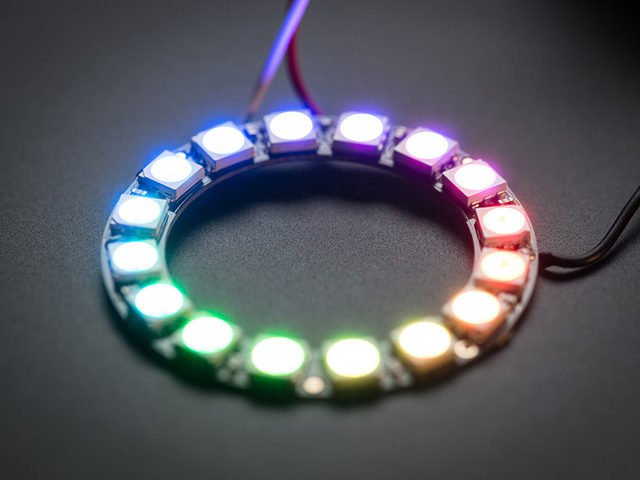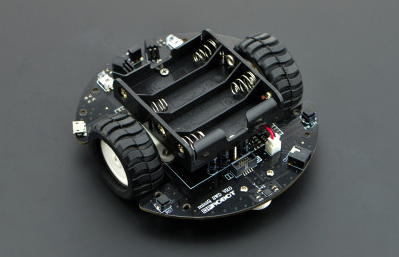ArduinoNeoPixelLine Following RobotArduino Basics

1. Programs are called sketches and have an extension of .ino. They must be in a folder named the same as the file.

2. There are two functions in a sketch:
setup() – This function is called when a sketch starts. Use it to initialize pin modes, serial communications, start using libraries, etc.

loop() – After creating a setup() function, which initializes and sets the initial values, the loop() function loops forever.  Use this for the main loop of your sketch (program).

3.  Once a program/sketch has been uploaded to an Arduino, it stays on it until a new one is loaded.  The board can be powered by a battery after the sketch is loaded.

4.  Tips
- Under Tools menu, check that Board is set to “Arduino/Genuino Uno”.  The MiniQ should be set to "Arduino Leonardo".
- Under Help menu, select Reference to see all the commands
- Under File menu, look at some the Examples

5.  Pseudo Random Numbers
Seed random # generator in setup:
Generate a random # between 0 and 9:
random(10);

6.  Serial Monitor
This allows you to send data from your board back to your computer. Click on the Serial Monitor icon on the right side of the toolbar.
Initialize serial monitor in setup():
Serial.begin(9600);

Output text:
Serial.println("Hello World!");
Output numbers/variables:
Serial.println(A);

 Sample NeoPixel Ring Code #include int NumPixels = 16; Adafruit_NeoPixel pixels = Adafruit_NeoPixel(NumPixels, 7);  // NumPixels, Pin#  // setup() runs once at the beginning void setup() {    pixels.begin(); // Initialize the NeoPixels    for (int n=0; n

 Sample Line Following Robot Code #include // Arduino Mega - connect QTR wires to Analog pins 0 - 7 QTRSensorsRC qtr((char[]) {54, 55, 56, 57, 58, 59, 60, 61}, 8); // Mega code // Arduino Uno - connect QTR wires to Analog pins 0 - 5 // QTRSensorsRC qtr((char[]) {14, 15, 16, 17, 18, 19}, 6); // Uno code unsigned int sensor; // connect motor controller pins to Arduino digital pins int enA = 2; int in1 = 3; int in2 = 4;   // motor 1 int in3 = 5; int in4 = 6; int enB = 7;   // motor 2 void Stop() {    digitalWrite(in1, LOW);    digitalWrite(in2, LOW);    digitalWrite(in3, LOW);    digitalWrite(in4, LOW); } void Forward (int LeftSpeed, int RightSpeed) // range 0 - 255 {    digitalWrite(in1, HIGH); // turn on left motor to forward    digitalWrite(in2, LOW);    analogWrite(enA, LeftSpeed);    digitalWrite(in3, HIGH); // turn on right motor to forward    digitalWrite(in4, LOW);    analogWrite(enB, RightSpeed); }void Reverse (int LeftSpeed, int RightSpeed) // range 0-255 {    digitalWrite(in1, LOW); // turn on left motor to forward    digitalWrite(in2, HIGH);    analogWrite(enA, LeftSpeed);    digitalWrite(in3, LOW); // turn on right motor to forward    digitalWrite(in4, HIGH);    analogWrite(enB, RightSpeed); } void setup() {    // set all the motor control pins to outputs    pinMode(enA, OUTPUT);    pinMode(enB, OUTPUT);    pinMode(in1, OUTPUT);    pinMode(in2, OUTPUT);    pinMode(in3, OUTPUT);    pinMode(in4, OUTPUT);       Serial.begin(9600); // used to write to serial monitor    // pushbutton    pinMode(53,INPUT_PULLUP);    while (digitalRead(53) != LOW) // wait for pushbutton to start motors    {       //for testing print out sensor value to Serial Monitor       //qtr.read(sensor);       //Serial.print(sensor); Serial.print(" "); Serial.print(sensor); Serial.print(" ");       //Serial.print(sensor); Serial.print(" "); Serial.print(sensor); Serial.print(" ");       //Serial.print(sensor); Serial.print(" "); Serial.print(sensor); Serial.print(" ");       //Serial.print(sensor); Serial.print(" "); Serial.print(sensor); Serial.print("\n");       //delay(500);    }    Forward(100,100); } void loop() {    qtr.read(sensor);    if (sensor<700)    {       Forward(40,200);    }    else if (sensor<700)    {       Forward(80,200);    }    else if (sensor<700)    {       Forward(100,200);    }    else if (sensor<700)    {       Forward(200,100);    }    else if (sensor<700)    {       Forward(200,80);    }    else if (sensor<700)    {       Forward(200,40);    }    else if (sensor < 700 || sensor < 700)    {       Forward(255,255);    }    else       Forward(150,150);    delay(30); }